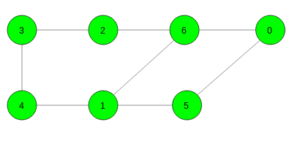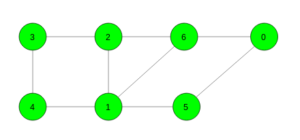# Shortest cycle in an undirected unweighted graph

Given an undirected unweighted graph. The task is to find the length of the shortest cycle in the given graph. If no cycle exists print -1.

Examples:

Input:Output: 4
Cycle 6 -> 1 -> 5 -> 0 -> 6

Input:Output: 3
Cycle 6 -> 1 -> 2 -> 6

## Recommended: Please try your approach on {IDE} first, before moving on to the solution.

Prerequisites: Dijikstra

Approach: For every vertex, we check if it is possible to get the shortest cycle involving this vertex. For every vertex first, push current vertex into the queue and then it’s neighbours and if vertex which is already visited comes again then the cycle is present.

Apply the above process for every vertex and get the length of the shortest cycle.

Below is the implementation of the above approach:

## C++

 `// C++ implementation of the approach ` `#include ` `using` `namespace` `std; ` `#define N 100200 ` ` `  `vector<``int``> gr[N]; ` ` `  `// Function to add edge ` `void` `Add_edge(``int` `x, ``int` `y) ` `{ ` `    ``gr[x].push_back(y); ` `    ``gr[y].push_back(x); ` `} ` ` `  `// Function to find the length of ` `// the shortest cycle in the graph ` `int` `shortest_cycle(``int` `n) ` `{ ` `    ``// To store length of the shortest cycle ` `    ``int` `ans = INT_MAX; ` ` `  `    ``// For all vertices ` `    ``for` `(``int` `i = 0; i < n; i++) { ` ` `  `        ``// Make distance maximum ` `        ``vector<``int``> dist(n, (``int``)(1e9)); ` ` `  `        ``// Take a imaginary parent ` `        ``vector<``int``> par(n, -1); ` ` `  `        ``// Distance of source to source is 0 ` `        ``dist[i] = 0; ` `        ``queue<``int``> q; ` ` `  `        ``// Push the source element ` `        ``q.push(i); ` ` `  `        ``// Continue until queue is not empty ` `        ``while` `(!q.empty()) { ` ` `  `            ``// Take the first element ` `            ``int` `x = q.front(); ` `            ``q.pop(); ` ` `  `            ``// Traverse for all it's childs ` `            ``for` `(``int` `child : gr[x]) { ` ` `  `                ``// If it is not visited yet ` `                ``if` `(dist[child] == (``int``)(1e9)) { ` ` `  `                    ``// Increase distance by 1 ` `                    ``dist[child] = 1 + dist[x]; ` ` `  `                    ``// Change parent ` `                    ``par[child] = x; ` ` `  `                    ``// Push into the queue ` `                    ``q.push(child); ` `                ``} ` ` `  `                ``// If it is already visited ` `                ``else` `if` `(par[x] != child and par[child] != x) ` `                    ``ans = min(ans, dist[x] + dist[child] + 1); ` `            ``} ` `        ``} ` `    ``} ` ` `  `    ``// If graph contains no cycle ` `    ``if` `(ans == INT_MAX) ` `        ``return` `-1; ` ` `  `    ``// If graph contains cycle ` `    ``else` `        ``return` `ans; ` `} ` ` `  `// Driver code ` `int` `main() ` `{ ` `    ``// Number of vertices ` `    ``int` `n = 7; ` ` `  `    ``// Add edges ` `    ``Add_edge(0, 6); ` `    ``Add_edge(0, 5); ` `    ``Add_edge(5, 1); ` `    ``Add_edge(1, 6); ` `    ``Add_edge(2, 6); ` `    ``Add_edge(2, 3); ` `    ``Add_edge(3, 4); ` `    ``Add_edge(4, 1); ` ` `  `    ``// Function call ` `    ``cout << shortest_cycle(n); ` ` `  `    ``return` `0; ` `} `

## Java

 `// Java timplementation of the approach  ` `import` `java.util.*; ` ` `  `class` `GFG  ` `{ ` ` `  `    ``static` `final` `int` `N = ``100200``; ` `    ``@SuppressWarnings``(``"unchecked"``) ` `    ``static` `Vector[] gr = ``new` `Vector[N]; ` ` `  `    ``// Function to add edge ` `    ``static` `void` `Add_edge(``int` `x, ``int` `y) ` `    ``{ ` `        ``gr[x].add(y); ` `        ``gr[y].add(x); ` `    ``} ` ` `  `    ``// Function to find the length of ` `    ``// the shortest cycle in the graph ` `    ``static` `int` `shortest_cycle(``int` `n) ` `    ``{ ` ` `  `        ``// To store length of the shortest cycle ` `        ``int` `ans = Integer.MAX_VALUE; ` ` `  `        ``// For all vertices ` `        ``for` `(``int` `i = ``0``; i < n; i++) ` `        ``{ ` ` `  `            ``// Make distance maximum ` `            ``int``[] dist = ``new` `int``[n]; ` `            ``Arrays.fill(dist, (``int``) 1e9); ` ` `  `            ``// Take a imaginary parent ` `            ``int``[] par = ``new` `int``[n]; ` `            ``Arrays.fill(par, -``1``); ` ` `  `            ``// Distance of source to source is 0 ` `            ``dist[i] = ``0``; ` `            ``Queue q = ``new` `LinkedList<>(); ` ` `  `            ``// Push the source element ` `            ``q.add(i); ` ` `  `            ``// Continue until queue is not empty ` `            ``while` `(!q.isEmpty()) ` `            ``{ ` ` `  `                ``// Take the first element ` `                ``int` `x = q.poll(); ` ` `  `                ``// Traverse for all it's childs ` `                ``for` `(``int` `child : gr[x]) ` `                ``{ ` `                    ``// If it is not visited yet ` `                    ``if` `(dist[child] == (``int``) (1e9)) ` `                    ``{ ` ` `  `                        ``// Increase distance by 1 ` `                        ``dist[child] = ``1` `+ dist[x]; ` ` `  `                        ``// Change parent ` `                        ``par[child] = x; ` ` `  `                        ``// Push into the queue ` `                        ``q.add(child); ` `                    ``} ``else` `if` `(par[x] != child && par[child] != x) ` `                        ``ans = Math.min(ans, dist[x] + dist[child] + ``1``); ` `                ``} ` `            ``} ` `        ``} ` ` `  `        ``// If graph contains no cycle ` `        ``if` `(ans == Integer.MAX_VALUE) ` `            ``return` `-``1``; ` ` `  `        ``// If graph contains cycle ` `        ``else` `            ``return` `ans; ` `    ``} ` ` `  `    ``// Driver Code ` `    ``public` `static` `void` `main(String[] args) ` `    ``{ ` ` `  `        ``for` `(``int` `i = ``0``; i < N; i++) ` `            ``gr[i] = ``new` `Vector<>(); ` ` `  `        ``// Number of vertices ` `        ``int` `n = ``7``; ` ` `  `        ``// Add edges ` `        ``Add_edge(``0``, ``6``); ` `        ``Add_edge(``0``, ``5``); ` `        ``Add_edge(``5``, ``1``); ` `        ``Add_edge(``1``, ``6``); ` `        ``Add_edge(``2``, ``6``); ` `        ``Add_edge(``2``, ``3``); ` `        ``Add_edge(``3``, ``4``); ` `        ``Add_edge(``4``, ``1``); ` ` `  `        ``// Function call ` `        ``System.out.println(shortest_cycle(n)); ` `    ``} ` `} ` ` `  `// This code is contributed by ` `// sanjeev2552 `

## Python3

 `# Python3 implementation of the approach ` `from` `sys ``import` `maxsize as INT_MAX ` `from` `collections ``import` `deque ` ` `  `N ``=` `100200` ` `  `gr ``=` `[``0``] ``*` `N ` `for` `i ``in` `range``(N): ` `    ``gr[i] ``=` `[] ` ` `  `# Function to add edge ` `def` `add_edge(x: ``int``, y: ``int``) ``-``> ``None``: ` `    ``global` `gr ` `    ``gr[x].append(y) ` `    ``gr[y].append(x) ` ` `  `# Function to find the length of ` `# the shortest cycle in the graph ` `def` `shortest_cycle(n: ``int``) ``-``> ``int``: ` ` `  `    ``# To store length of the shortest cycle ` `    ``ans ``=` `INT_MAX ` ` `  `    ``# For all vertices ` `    ``for` `i ``in` `range``(n): ` ` `  `        ``# Make distance maximum ` `        ``dist ``=` `[``int``(``1e9``)] ``*` `n ` ` `  `        ``# Take a imaginary parent ` `        ``par ``=` `[``-``1``] ``*` `n ` ` `  `        ``# Distance of source to source is 0 ` `        ``dist[i] ``=` `0` `        ``q ``=` `deque() ` ` `  `        ``# Push the source element ` `        ``q.append(i) ` ` `  `        ``# Continue until queue is not empty ` `        ``while` `q: ` ` `  `            ``# Take the first element ` `            ``x ``=` `q[``0``] ` `            ``q.popleft() ` ` `  `            ``# Traverse for all it's childs ` `            ``for` `child ``in` `gr[x]: ` ` `  `                ``# If it is not visited yet ` `                ``if` `dist[child] ``=``=` `int``(``1e9``): ` ` `  `                    ``# Increase distance by 1 ` `                    ``dist[child] ``=` `1` `+` `dist[x] ` ` `  `                    ``# Change parent ` `                    ``par[child] ``=` `x ` ` `  `                    ``# Push into the queue ` `                    ``q.append(child) ` ` `  `                ``# If it is already visited ` `                ``elif` `par[x] !``=` `child ``and` `par[child] !``=` `x: ` `                    ``ans ``=` `min``(ans, dist[x] ``+`  `                                   ``dist[child] ``+` `1``) ` ` `  `    ``# If graph contains no cycle ` `    ``if` `ans ``=``=` `INT_MAX: ` `        ``return` `-``1` ` `  `    ``# If graph contains cycle ` `    ``else``: ` `        ``return` `ans ` ` `  `# Driver Code ` `if` `__name__ ``=``=` `"__main__"``: ` ` `  `    ``# Number of vertices ` `    ``n ``=` `7` ` `  `    ``# Add edges ` `    ``add_edge(``0``, ``6``) ` `    ``add_edge(``0``, ``5``) ` `    ``add_edge(``5``, ``1``) ` `    ``add_edge(``1``, ``6``) ` `    ``add_edge(``2``, ``6``) ` `    ``add_edge(``2``, ``3``) ` `    ``add_edge(``3``, ``4``) ` `    ``add_edge(``4``, ``1``) ` ` `  `    ``# Function call ` `    ``print``(shortest_cycle(n)) ` ` `  `# This code is contributed by ` `# sanjeev2552 `

## C#

 `// C# timplementation of the approach  ` `using` `System; ` `using` `System.Collections.Generic; ` ` `  `class` `GFG  ` `{  ` ` `  `    ``static` `readonly` `int` `N = 100200;  ` `    ``static` `List<``int``>[] gr = ``new` `List<``int``>[N];  ` ` `  `    ``// Function to add edge  ` `    ``static` `void` `Add_edge(``int` `x, ``int` `y)  ` `    ``{  ` `        ``gr[x].Add(y);  ` `        ``gr[y].Add(x);  ` `    ``}  ` ` `  `    ``// Function to find the length of  ` `    ``// the shortest cycle in the graph  ` `    ``static` `int` `shortest_cycle(``int` `n)  ` `    ``{  ` ` `  `        ``// To store length of the shortest cycle  ` `        ``int` `ans = ``int``.MaxValue;  ` ` `  `        ``// For all vertices  ` `        ``for` `(``int` `i = 0; i < n; i++)  ` `        ``{  ` ` `  `            ``// Make distance maximum  ` `            ``int``[] dist = ``new` `int``[n];  ` `            ``fill(dist, (``int``) 1e9);  ` ` `  `            ``// Take a imaginary parent  ` `            ``int``[] par = ``new` `int``[n];  ` `            ``fill(par, -1);  ` ` `  `            ``// Distance of source to source is 0  ` `            ``dist[i] = 0;  ` `            ``List<``int``> q = ``new` `List<``int``>();  ` ` `  `            ``// Push the source element  ` `            ``q.Add(i);  ` ` `  `            ``// Continue until queue is not empty  ` `            ``while` `(q.Count!=0)  ` `            ``{  ` ` `  `                ``// Take the first element  ` `                ``int` `x = q; ` `                ``q.RemoveAt(0);  ` ` `  `                ``// Traverse for all it's childs  ` `                ``foreach` `(``int` `child ``in` `gr[x])  ` `                ``{  ` `                    ``// If it is not visited yet  ` `                    ``if` `(dist[child] == (``int``) (1e9))  ` `                    ``{  ` ` `  `                        ``// Increase distance by 1  ` `                        ``dist[child] = 1 + dist[x];  ` ` `  `                        ``// Change parent  ` `                        ``par[child] = x;  ` ` `  `                        ``// Push into the queue  ` `                        ``q.Add(child);  ` `                    ``} ``else` `if` `(par[x] != child && par[child] != x)  ` `                        ``ans = Math.Min(ans, dist[x] + dist[child] + 1);  ` `                ``}  ` `            ``}  ` `        ``}  ` ` `  `        ``// If graph contains no cycle  ` `        ``if` `(ans == ``int``.MaxValue)  ` `            ``return` `-1;  ` ` `  `        ``// If graph contains cycle  ` `        ``else` `            ``return` `ans;  ` `    ``}  ` `    ``static` `int``[] fill(``int` `[]arr, ``int` `val) ` `    ``{ ` `        ``for``(``int` `i = 0;i();  ` ` `  `        ``// Number of vertices  ` `        ``int` `n = 7;  ` ` `  `        ``// Add edges  ` `        ``Add_edge(0, 6);  ` `        ``Add_edge(0, 5);  ` `        ``Add_edge(5, 1);  ` `        ``Add_edge(1, 6);  ` `        ``Add_edge(2, 6);  ` `        ``Add_edge(2, 3);  ` `        ``Add_edge(3, 4);  ` `        ``Add_edge(4, 1);  ` ` `  `        ``// Function call  ` `        ``Console.WriteLine(shortest_cycle(n));  ` `    ``}  ` `}  ` ` `  `// This code is contributed by 29AjayKumar `

Output:

```4
```

My Personal Notes arrow_drop_upCheck out this Author's contributed articles.

If you like GeeksforGeeks and would like to contribute, you can also write an article using contribute.geeksforgeeks.org or mail your article to contribute@geeksforgeeks.org. See your article appearing on the GeeksforGeeks main page and help other Geeks.

Please Improve this article if you find anything incorrect by clicking on the "Improve Article" button below.

Improved By : sanjeev2552, 29AjayKumar

Article Tags :
Practice Tags :

3

Please write to us at contribute@geeksforgeeks.org to report any issue with the above content.